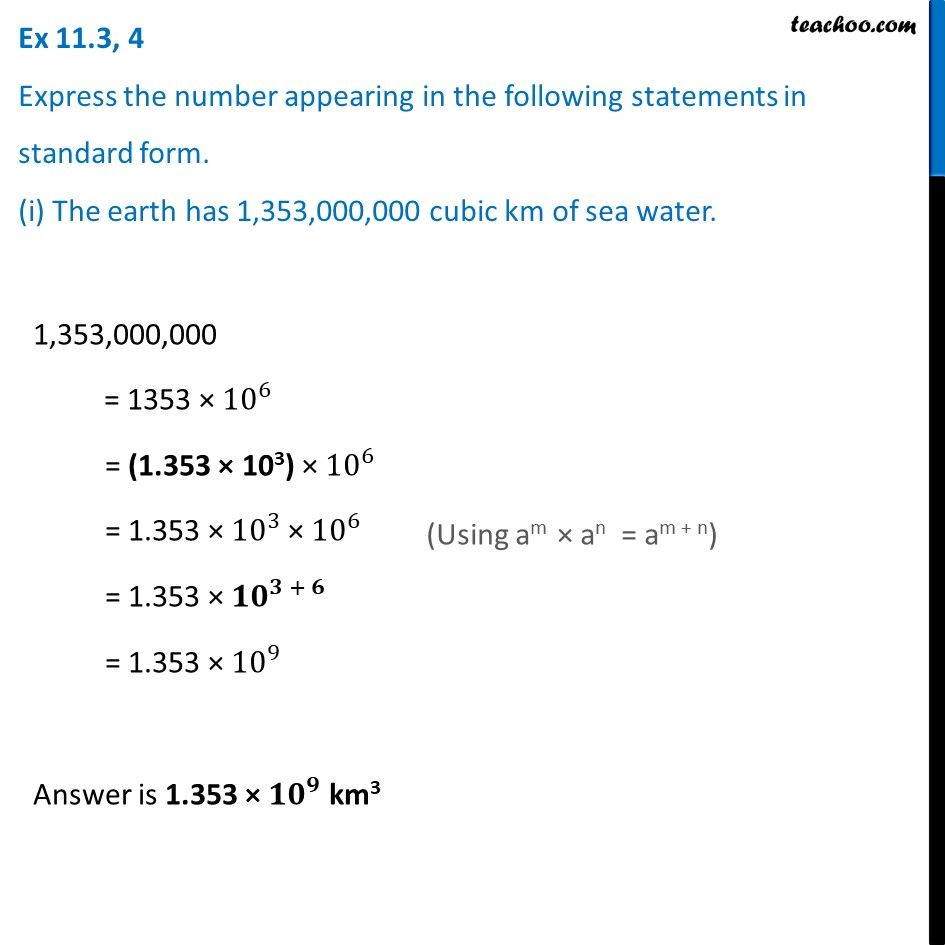Ex 11.3

Chapter 11 Class 7 Exponents and Powers
Serial order wiseLearn in your speed, with individual attention - Teachoo Maths 1-on-1 Class

### Transcript

Ex 11.3, 4 Express the number appearing in the following statements in standard form. (i) The earth has 1,353,000,000 cubic km of sea water. 1,353,000,000 = 1353 × 10^6 = (1.353 × 103) × 10^6 = 1.353 × 10^3 × 10^6 = 1.353 × 10^(3 + 6) = 1.353 × 10^9 Answer is 1.353 × 〖𝟏𝟎〗^𝟗 km3